# Find the wavelength, frequency and time period of the wave?

1. What is meant by frequency of sound waves?
2. Give the range of frequencies of sound waves that an average human ear can detect.
3. A source of wave produces 20 crests and 20 troughs in 0.2 s. The distance between a crest and next trough is 50 cm. Find the
• wavelength
• frequency
• time period of the wave

1.Frequency The number of waves produced per second is called the frequency of the wave
2. 20 Hz to 20 kHz
3.

• Since the distance between a crest and next trough is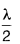Therefore,= 50 cm
⇒ λ = 100 cm or 1 m
• Distance covered in 20 crests = 20 x 1 = 20 m
∴ Velocity of the wave, v = vλ =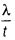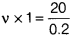[∵ t = 0.2 s]
∴ v = 100 Hz
or 1 crest and 1 through = 1 waves
∴ 20 crests and 20 throughs = 20 waves
∴ Frequency =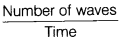=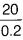= 100 Hz
• ∴ Time period, T ==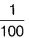= 0.01 s